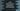# Java Math signum function explanation with examples## Java Math signum function explanation with examples:

Let’s learn how to use the signum function in Java with examples. The signum method is used to get the signum function of the argument. It can take a float or double value and returns float or double.

In this post, I will show you how signum function works with its definition and examples.

### Definition of signum:

The signum function is defined as like below:

``public static double signum(double d)``

And,

``public static float signum(float f)``

As you can see here,

• It is a static method, i.e. we can call it directly in the Math class.
• It returns the signum function of the given argument.

• If the argument is zero, it will return zero. If it is greater than zero, it will return 1.0, and if it is less than zero, it will return -1.0.
• If the argument is NaN, it will return NaN.
• If the argument is positive zero, it will return positive zero. If it is negative zero, it will return negative zero.

### Return value of signum:

The signum function returns a floating point value or double value based on the parameter type. The return value type is same as the parameter value.

### Example of signum with float:

Let’s try signum with different floating point variables:

``````class Main {
public static void main(String[] args) {
float[] numArray = {10.0f, -12.0f, 0.0f, 0f, 13.3f};

for (float i : numArray) {
System.out.println(i + " => " + Math.signum(i));
}

}
}``````

In this program, we initialized an array with float values and using Math.signum on each value in this array. The for loop iterates through the elements of the array and prints its value along with the result of Math.signum.

If you run the above program, it will print the below output:

``````10.0 => 1.0
-12.0 => -1.0
0.0 => 0.0
0.0 => 0.0
13.3 => 1.0``````

You can see here that it returns -1.0 for all negative numbers, 1.0 for all positive numbers and 0.0 for zero.

Let’s try it with doubles:

### Example of signum with double:

I am changing the above program to use double values instead of float:

``````class Main {
public static void main(String[] args) {
double[] numArray = {10.012333, -12.0, 0.0, 0, 13.3};

for (double i : numArray) {
System.out.println(i + " => " + Math.signum(i));
}

}
}``````

If you run this program, it will print the below output:

``````10.0 => 1.0
-12.0 => -1.0
0.0 => 0.0
0.0 => 0.0
13.3 => 1.0``````

The result is similar to the above one, but the return type is different. These are all double values.